# David Kohel's Course

## MSRI 2006 Summer Graduate Workshop in Computational Number Theory

 Below is a sketch of topics that are of interest to me, at various inconsistent levels of depth. I omitted "modular forms", which William will cover in great depth, but mentioned CM, which wasn't assigned to anyone, and Siegel/Hilbert modular forms which Lassina and Paul will presumably cover. I thought that some of the complex analytic approaches might be suitable to computation, and fun to implement. Although I call `G\H^*' a Riemann surface, I am thinking of this as a widget for invariants of the group action of G on H. As a Riemann surface, however, one could ask for the image of a special point (i.e. its equivalence class), and create divisors, integrate along paths, etc. It would be easy to stretch two weeks into a semester, so of course we need to decide on limitations of the scope. I will probably only cover 1 and 3; although 2 and 4 would be desirable (very classical stuff). Item 5 would probably fit better in the framework of Lassina's talks (see his comment after his additional topic 6)).1.
Arithmetic of quaternion algebras.
1. Arithmetic of algebras, orders, and ideals.
1. Algebras over global fields.
2. Local arithmetic of quaternion algebras.
3. Local to global correspondence.
4. Brauer groups.
5. Ideles, lattices, and class groups.
2. Computational aspects of quaternion algebras.
1. Construction of quaternion algebras with prescribed ramification.
2. Relations with quadratic forms, conics, local Hilbert symbols, and Hasse-Minkowski.
3. Galois cohomology and reprsentations of Brauer classes.
4. Structures of lattices, orders, and ideals; left and right orders and neighboring constructions.
Project: Arithmetic of quaternion algebras.
2.
Discrete groups and quotients of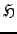.
1. Fuchsian subgroups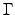of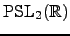.
2. Hyperbolic geometry of, and invariants of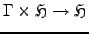3. Genus of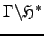.
4. CM lattices and class groups.
Project: Classical modular curves (and Shimura curves) as Riemann surfaces; associated invariants of, integration of modular forms and period lattices (combining with lectures on modular forms) and complex homology.
3.
Brandt modules.
Refer to Pizer, Kohel (article and unpublished preprint), Lassina-Kohel-Stein; relation with Mestre's Méthode des graphes, and Birch's ternary forms method.
Project: Brandt modules implementation: extensions to weight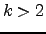, experiment with different hashing invariants, etc.; effective isomorphism with supersingular methods of graphs, and Birch's method.
4.
Complex multiplication.
Lectures on complex multiplication in dimension 1 and dimension g?
Project: Arithmetic of binary quadratic forms and modular class invariants for CM divisors; points in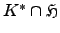and path maps to complex homology groups.
5.
Siegel and Hilbert modular forms.
Lectures on Siegel and Hilbert modular forms.
Project: Hyperbolic geometry of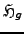and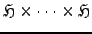(with given real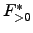structure), with focus on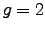and and embeddings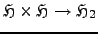; Shimura curves on these surfaces.